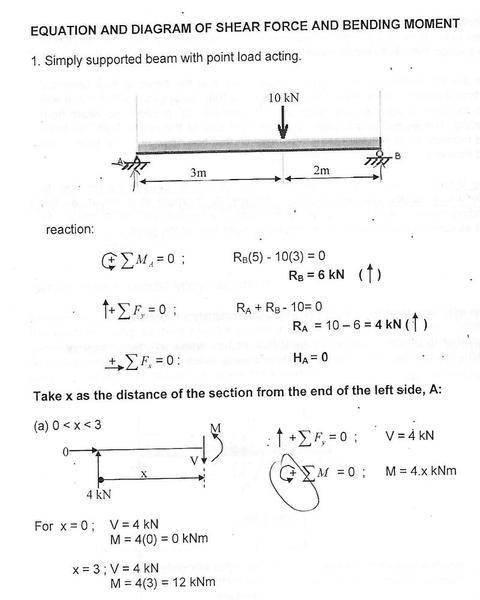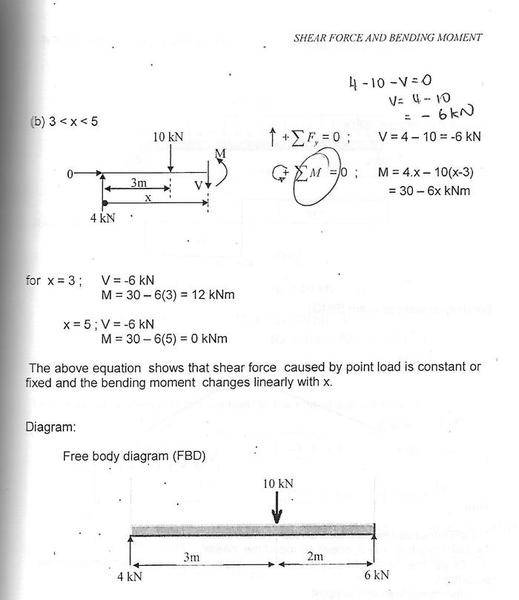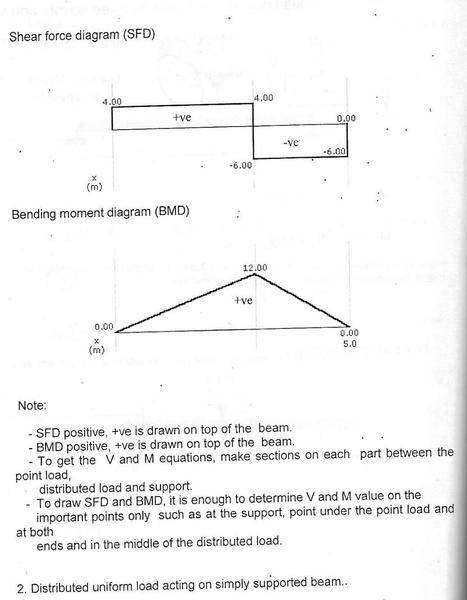# Direction of shearing force

## Homework Statement

why for 0 <x <3 , why the author ignore the 10kN ? as we can see , the 10kN is located 3m from A ......
2nd question is , the author assume downwards force is positive , am i right ?## The Attempt at a Solution

for 0 <x <3 , the shearing force V = 4kN , as we can can see , at A the support force (4kN) is pointing upwards , so the shearing force 4kN should be pointing downwards , am i right ? so , the author assume downwards force is positive??
if so , why in the shearing force diagram (SFD) , the 4kN is at positive x direction , shouldn't it at 4kN bottom of the x-axis ?

one more question , why the moment at 3 <x<5 is 4x - 10(x-3) , the author taking moment at which point ?

Make an imaginary cut in the beam. If the shear force is pointing up to the left of the cut then shear force is positive by convention.

Everything to the right of the cut we ignore (for purposes of calculating shear force) because we know beam is in equilibrium. That means you can automatically assume whatever shear force (or bending moment) exists on the left face of the cut, it is equal & opposite to that which exists on the right face.

Last edited:
Make an imaginary cut in the beam. If the shear force is pointing up to the left of the cut then shear force is positive by convention.

Everything to the right of the cut we ignore (for purposes of calculating shear force) because we know beam is in equilibrium. That means you can automatically assume whatever shear force (or bending moment) exists on the left face of the cut, it is equal & opposite to that which exists on the right face.
Why??

Because if internal forces are not equal & opposite, the beam will fail. Any imaginary slice will render mirror image shear, torsion, tension and compression forces between the left and right faces of that section.